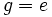# From cohomology to representation theory

This is a survey article still in the making, and may contain some factual or conceptual errors. Please take it with a pinch of salt

## What is cohomology and what is representation theory

### Cohomology

Given a topological space (or a manifold, or an algebraic variety) we are sometimes able to assiciate with that a chain complex of Abelian groups. We can then look at functions from the Abelian groups in this chain complex, to a certain ring, and use these functions to obtain a dual, cochain complex.

Cohomology is about studying the homology of this complex (in other words, the quotient of the kernel of each map by the image of the previous map).

### Representation theory

We are given a group (any group, may be finite or infinite). The representation theory of this group is the story of homomorphisms from the group to general linear groups of vector spaces over fields. Equivalently, it is about the theory of modules over the group ring of the given group over a given field.

The representation theory of a group$G$ over a field$k$, is defined as the theory of all homomorphisms$\rho: G \to GL(V)$ where$V$ is a$k$-vector space. Equivalently, it is the theory of all$kG$-modules, viz modules over the group ring of$G$ over$k$.

When we use the group ring language, we can generalize representation theory to work over any ring. The representation theory of a group$G$ over a ring$R$ is the story of$RG$-modules.

## Why they may be related

Suppose a group$G$ acts on a space$X$. This may be:

•$X$ is a topological space and$G$ acts as self-homeomorphisms of$X$
•$X$ is a differential manifold and$G$ acts as self-diffeomorphisms of$X$
•$X$ is an algebraic variety and$G$ acts as algebraic automorphisms of$X$

Then, for any$g \in G$, we have an automorphism of$X$ (which may be a self-homeomorphism, self-diffeomorphism, or algebraic automorphism). By the functoriality of cohomology, each$g \in G$ induces a map from$H^i(X)$ to itself (for every$i$). If our cohomology theory is with coefficients in$R$, the induced map is an$R$-module map.

Thus, for every$i$, we have a map from$G$ to the set of$R$-module automorphisms of$H^i(X)$. This map thus defines a group action of$G$ on the$R$-module$H^i(X)$, and hence, makes$H^i(X)$ into a$RG$-module. Thus, we obtain a representation of$G$.

The upshot:

An action of a group on a space, gives a family of representations of the group over any base ring, via the use of a cohomology theory of the space with coefficients in that base ring.

## Some particular simple cases

### For a discrete set of points

The cohomology theory of a discrete finite set of points has, as its first cohomology group, the free module over the base ring with rank equal to the number of points. Later cohomology groups are trivial.

Thus, any permutation representation over the finite set of points gives a representation on the free module on that many points. This, incidentally, is the same as the original permutation representation, viewing the permutations as matrices.

Thus, in some sense, the passage from actions on spaces, to representations over rings, is a generalization of the passage from a permutation representation to the associated linear representation.

### For a finite simplicial complex

For a simplicial complex, we can use simplicial cohomology.

## Trace formulae

A favourite tool of cohomology theorists is to take signed summations, and then to say that the quantities obtained by the signed summations are some sort of invariant. We do look at various signed summations, typically of:

• The characters of representations, varying over$H^i$ but keeping an element$g \in G$ constant. Remember that the character of a representation is the same as the trace, when written in a suitable matrix form (we can make sense of all these notions when the modules are all free)
• The dimensions of the representations (which is really a special case of the above, setting$g = e$)

Important instances of such trace formulae (also called character formulae) are: